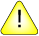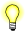This version of this document is no longer maintained. For the latest documentation, see http://www.qnx.com/developers/docs.

# pow(), powf()

Raise a number to a given power

## Synopsis:

```#include <math.h>

double pow( double x,
double y );

float powf( float x,
float y );```

## Arguments:

x
The number you want to raise.
y
The power you want to raise the number to.

## Library:

libm

Use the -l m option to qcc to link against this library.

## Description:

The pow() and powf() functions compute x raised to the power of y.

A domain error occurs if x = 0, and y <= 0, or if x is negative, and y isn't an integer. A range error may also occur.

## Returns:

The value of xy.If an error occurs, these functions return 0, but this is also a valid mathematical result. If you want to check for errors, set errno to 0, call the function, and then check errno again. These functions don't change errno if no errors occurred.

## Examples:

```#include <stdio.h>
#include <stdlib.h>
#include <math.h>

int main( void )
{
printf( "%f\n", pow( 1.5, 2.5 ) );

return EXIT_SUCCESS;
}```

produces the output:

`2.755676`

## Classification:

Safety:
Cancellation point No
Interrupt handler No
Signal handler No